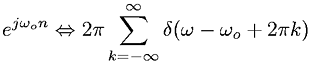Equations > Signal Processing > Fourier Transform Pairs > Discrete-Time Fourier transform of complex exponential

### Discrete-Time Fourier transform of complex exponentialLatex Code:

MathML Code:

 ${e}^{j{\omega }_{o}n}⇔2\pi \sum _{k=-\infty }^{\infty }\delta \left(\omega -{\omega }_{o}+2\pi k\right)$

MathType 5.0: